# ISEE Lower Level Quantitative : How to find the decimal equivalent of a fraction

## Example Questions

### Example Question #11 : Fractions

What is the decimal equivalent of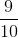?

Possible Answers: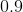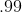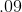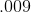Correct answer:Explanation:

To find the decimal equivalent, divide the numerator by denominator. Divide 9 by 10. Your result is 0.9.

### Example Question #11 : How To Find The Decimal Equivalent Of A Fraction

Write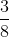as a decimal.

Possible Answers: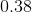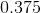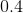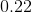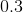Correct answer:Explanation:

Divide 3 by 8 as follows: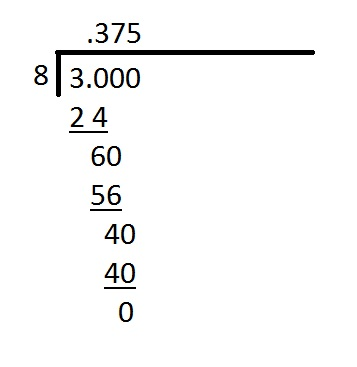The quotient, and decimal equivalent, is 0.375.

### Example Question #11 : Fractions

Write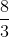as a decimal.

Possible Answers: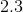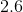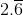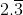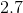Correct answer:Explanation:

Divide 8 by 3 as follows: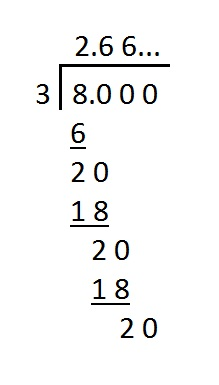It can be seen that the digit "6" repeats forever. Therefore, the quotient, and the decimal equivalent, is.

### Example Question #13 : How To Find The Decimal Equivalent Of A Fraction

Write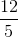as a decimal.

Possible Answers: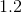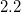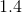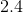Correct answer:Explanation:

Divide 12 by 5 as follows: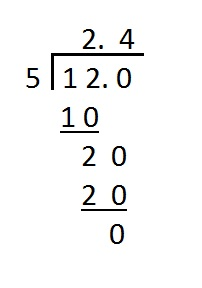2.4 is the quotient, and the decimal equivalent.

### Example Question #12 : Fractions

Write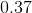as a fraction in simplest form.

Possible Answers: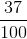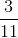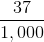Correct answer:Explanation:

The expression means "thirty-seven hundredths", so write.

Since 37 and 100 have a greatest common factor of 1, the fraction cannot be simplified further.

### Example Question #601 : Isee Lower Level (Grades 5 6) Quantitative Reasoning

Which of the following can fill in the blank to make the sentence below true?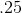is ____.

Possible Answers:

less than

more than

twice the value of

equal to

Correct answer:

less than

Explanation:is less than. Specifically, .25 is one third of. Therefore, the correct answer is "less than."

### Example Question #11 : Fractions

Write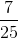as a decimal.

Possible Answers: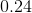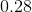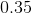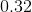Correct answer:Explanation:

Divide 7 by 25 as follows: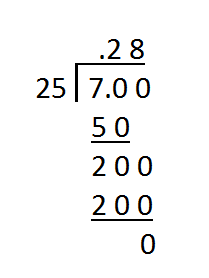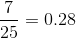### Example Question #12 : Fractions

Write 0.72 as a fraction in lowest terms.

Possible Answers: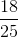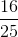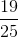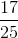Correct answer:Explanation:

The expression means "seventy-two one hundredths", so we initially write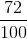The greatest common factor of the numerator and the denominator is equal to 4, so reduce as follows: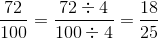### Example Question #602 : Isee Lower Level (Grades 5 6) Quantitative Reasoning

How can the number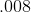be written in words?

Possible Answers:

Eight hendredths

Eight ten thousandths

Eight thousands

Eight thousandths

Correct answer:

Eight thousandths

Explanation:

Given that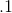is equal to one tenth,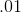is equal to one hundredth, and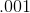is equal to one thousandth, it follows thatis equal to eight thousandths.

### Example Question #11 : How To Find The Decimal Equivalent Of A Fraction

Write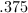as a fraction in simplest form.

Possible Answers: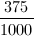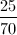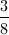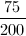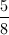Correct answer:Explanation:

In order to writeas a fraction in the most simple form, we must first write it as a fraction.Now we must divide the numerator and denominator by the greatest common factor, which in this case is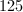.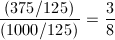### All ISEE Lower Level Quantitative Resources